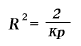Albert Einstein: Relativity
Part III: Considerations on the Universe as a Whole

### The Structure of Space According to the General Theory of Relativity

According to the general theory of relativity, the geometrical properties of space are not independent, but they are determined by matter. Thus we can draw conclusions about the geometrical structure of the universe only if we base our considerations on the state of the matter as being something that is known. We know from experience that, for a suitably chosen co-ordinate system, the velocities of the stars are small as compared with the velocity of transmission of light. We can thus as a rough approximation arrive at a conclusion as to the nature of the universe as a whole, if we treat the matter as being at rest.

We already know from our previous discussion that the behaviour of measuring-rods and clocks is influenced by gravitational fields, i.e. by the distribution of matter. This in itself is sufficient to exclude the possibility of the exact validity of Euclidean geometry in our universe. But it is conceivable that our universe differs only slightly from a Euclidean one, and this notion seems all the more probable, since calculations show that the metrics of surrounding space is influenced only to an exceedingly small extent by masses even of the magnitude of our sun. We might imagine that, as regards geometry, our universe behaves analogously to a surface which is irregularly curved in its individual parts, but which nowhere departs appreciably from a plane: something like the rippled surface of a lake. Such a universe might fittingly be called a quasi-Euclidean universe. As regards its space it would be infinite. But calculation shows that in a quasi-Euclidean universe the average density of matter would necessarily be nil. Thus such a universe could not be inhabited by matter everywhere ; it would present to us that unsatisfactory picture which we portrayed in Section 30.

If we are to have in the universe an average density of matter which differs from zero, however small may be that difference, then the universe cannot be quasi-Euclidean. On the contrary, the results of calculation indicate that if matter be distributed uniformly, the universe would necessarily be spherical (or elliptical). Since in reality the detailed distribution of matter is not uniform, the real universe will deviate in individual parts from the spherical, i.e. the universe will be quasi-spherical. But it will be necessarily finite. In fact, the theory supplies us with a simple connection 1) between the space-expanse of the universe and the average density of matter in it.

#### Footnotes

1) For the radius R of the universe we obtain the equationThe use of the C.G.S. system in this equation gives 2/k = 1.08.1027; p is the average density of the matter and k is a constant connected with the Newtonian constant of gravitation.